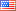Question-and-Answer Resource for the Building Energy Modeling Community
Get s tarted with the Help page

# Revision history [back]

### Air boundary condition

Hello, I wanted to ask a question about the air boundary condition. Does anybody know how the heat balance equation is written in a zone for the geometries, use air boundary? Is the temperature jump between two neighbor zones is reasonable? do we have convection between two neighbors, when we use the air boundary? I mainly want to know if the two neighbor zones are separated by air boundary material are assumed as two zones separated by fully transparent glass or separated by air jet?

Best, Sina Roozbahani

### Air boundary condition

Hello, I wanted to ask a question about the air boundary condition. condition. Does anybody know how the heat balance equation is written in a zone for the geometries, that use air boundary? Is the temperature jump between two neighbor zones is reasonable? do we have convection between two neighbors, when we use the air boundary? I mainly want to know if the two neighbor zones are separated by air boundary material are assumed as two zones separated by fully transparent glass or they are for example separated by air jet?

Best, Sina Roozbahani3 retaggedAaron Boranian12575 ●49 ●20 http://bigladdersoftware.com/

### Air boundary condition

Hello, I wanted to ask a question about the air boundary condition. Does anybody know how the heat balance equation is written in a zone for the geometries, that use air boundary? Is the temperature jump between two neighbor zones is reasonable? do we have convection between two neighbors, when we use the air boundary? I mainly want to know if the two neighbor zones are separated by air boundary material are assumed as two zones separated by fully transparent glass or they are for example separated by air jet?

Best, Sina Roozbahani

### Air boundary condition

Hello, I wanted to ask a question about the air boundary condition. Does anybody know how the heat balance equation is written in a zone for the geometries, that use air boundary? Is the temperature jump between two neighbor zones is reasonable? do we have convection between two neighbors, when we use the air boundary? I mainly want to know if the two neighbor zones are separated by air boundary material are assumed as two zones separated by fully transparent glass or they are for example separated by air jet?

Best, Sina Roozbahani

### Air boundary condition

Hello, I wanted to ask a question about the air boundary condition. Does anybody know how the heat balance equation is written in a zone for the geometries, that use air boundary? Is the temperature jump between two neighbor zones is reasonable? do we have convection between two neighbors, when we use the air boundary? I mainly want to know if the two neighbor zones are separated by air boundary material are assumed as two zones separated by fully transparent glass or they are for example separated by air jet?

Best, Sina Roozbahani